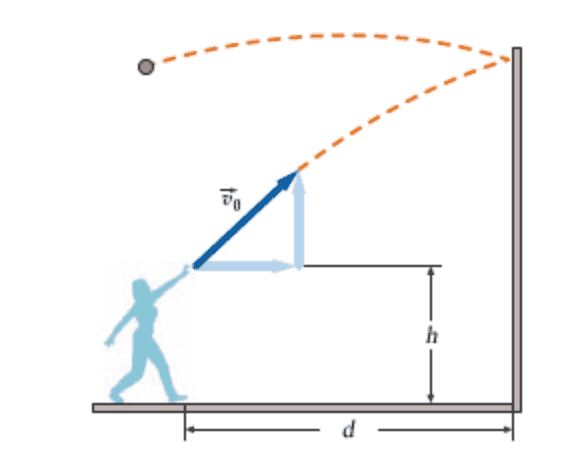# Throwing a ball against a wall for projectile motion

## Homework Statement

A woman throws a ball at a vertical wall d = 3.4 m away. The ball is h = 1.7 m above ground when it leaves the woman's hand with an initial velocity of 11 m/s at 45°. When the ball hits the wall, the horizontal component of its velocity is reversed; the vertical component remains unchanged. (Ignore any effects due to air resistance.)
(a) Where does the ball hit the ground? meters (from the wall)
(b) How long was the ball in the air before it hit the wall? seconds
(c) Where did the ball hit the wall? meters (above the ground)
(d) How long was the ball in the air after it left the wall? seconds## Homework Equations

gravity=-9.8m/s2
Time= 0=v0T-½gT2 T<0
t= (v0y+√(v0y2-2gy))/g
distance/range x=x0+V0xt
x/y velocity- v0x=Vcos(θ); v0y=vsin(θ)

## The Attempt at a Solution

I did this for a practice problem with different values, and got the correct answers. With these values it says i've gotten all incorrect answers… If someone could please help and verify what i've done wrong!

*I made the person's hand the origin
We're not dealing with forces, and therefor we don't lose velocity due to impact.

V0y= 11sin(45°)=7.778
Solving for total time t: ½(9.81)t2-7.778t-1.7 (h=1.7)
t=+1.324s

(a) I solved for total distance as regular, and subtracted (d=3.4)
v0x=11cos(45)=7.778
X=0+7.778(1.324)-3.4
x=10.29-3.4= 6.898m

(b) i made the x value 3.4 (distance)
3.4=0+7.778t
t=.539s

(c ) I plugged in the time it took to get to the wall to the vertical formula, and added h 1.7
y=7.778(5.39)-½(9.81)(5.39t2)+1.7
y=4.47m

(d)
total time 1.324 - ans(b) = .784s

Last edited:

## Answers and Replies

Simon Bridge
Science Advisor
Homework Helper
Solving for total time t: ½(9.81)t2-7.778t-1.7 (h=1.7)
Do you mean: $$\frac{1}{2}gT^2-vT\sin\theta-h=0$$ ... it can really help to keep the variables around as long as possible.

Did you realize that ##\sin(45)=\cos(45)=\frac{1}{\sqrt{2}}##?

The idea is to solve:
##x=\frac{1}{\sqrt{2}}vT - d## ... where x is the distance from the wall.

Where T is given by:
$$T=\frac{-v + \sqrt{v^2 +4gh}}{g\sqrt{2}}$$
... it's a + sign in there because we don't want to accept T<0.

So $$x=\frac{-v^2 + v\sqrt{v^2 +4gh}}{2g} -d$$

OK: if this looks like what you did, and you got it wrong, then the only thing I can think of is that you rounded something off somewhere or your calculator threw up a bogus value or something like that.

Check the arithmetic.

Also always possible that the model answer is incorrect.

•1 person
I actually found that I did my arithmetic wrong solving for time (subtracted instead of addition).

Thanks for the help!

Simon Bridge
Science Advisor
Homework Helper
Happens to the best of us :)